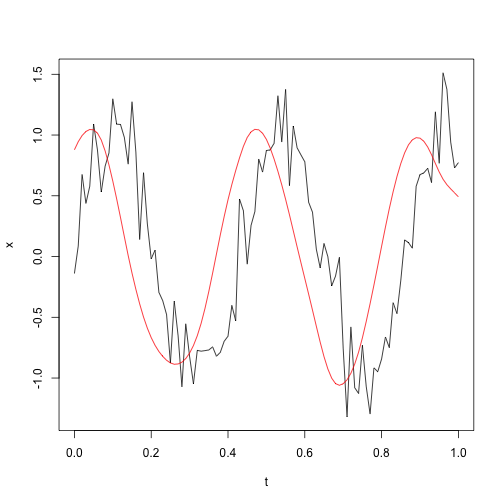The `filtfilt` function in R is supposedly based on the Matlab one, but it does quite badly on endpoints. My goal here is to explore alternatives, with a focus on the Octave method.

NOTE: I may also try scipy for hints.)

The best method of dealing with endpoints is still an open question. Matlab does one thing, Octave does another. Both seem to work reasonably well, and the Octave license is more appropriate to the present task, so I am focussing on that.

This is a page in progress. I am trying to make some of my R code work exactly as Octave does, since then I’ll know that I’ve successfully mimicked their method. This is proving to be difficult! I started this page weeks ago and have returned to it several times. If/when I figure it out, I will update the date of the blog posting and remove the “draft” designation.

# Octave implementation

## Test# R implementation# Comparison between R and octave output

The test codes a.m and a.R are the basis for the above.

Some R values (and comparisons with octave output) are given below.

• `si`: 0.9971, -1.3857, 0.535 (expect 0.99710 -1.38569 0.53497)
• `lrefl`: 9 (expect 9)
• `si_v1`: -1.1293, 1.5694, -0.6059` (expect -1.12926 1.56935 -0.60588)`
• `si_vend`: 0.8557, -1.1891, 0.4591` (expect 0.65164 -0.90559 0.34962)`
• `v_before_first_filter`: -1.1325, -1.0238, -0.8117, -1.1579 (expect -1.132546 -1.023766 -0.811650 -1.157909 -1.370316 -0.858954 -0.717951 …)
• `v_after_first_filter`: -0.6099, -0.4232, -0.219, -0.0097 (expect -1.157285 -1.123425 -1.072315 -1.001433 -0.909024 -0.794494 -0.658707 …)

Since v was ok before the filter, and wrong after, the problem seems to be in filter().

Finding the problem may be arduous, since (a) the R function `stats::filter()` is not well documented, regarding `init`, `init.x` and `init.y`, and (b) the Octave `filter()` spans 700 lines of C, so it is hard to reverse engineer to figure out what it’s doing.

## filter.cc

I’ll start by assuming that this is trying to mimic the Matlab function of the same name, although the 4th arg is called `si` in Octave and `zi` in Matlab, so some caution is warranted.

The docs on the matlab version are here

1. it takes 4 args, the last of which (`si`) is perhaps worth investigating, since it’s different from the initialization in the R filter (which has 3 items).

2. `si` gets renamed as `psi` in line 166

# Resources

• Matlab test code a.m
• R signal::filter.R
• R test code a.R
• test data used by above two codes x.dat
• matlab filtfilt.m
• the octave script filtfilt.m can be acquired by typing `pkg install -forge signal` in an Octave window, and extracting file named `~/octave/signal-1.3.0/filtfilt.m`
• the octave C filtering code filter.cc is the file `libinterp/corefcn/filter.cc` in the Octave source.

This website is written in Jekyll, and the source is available on GitHub.